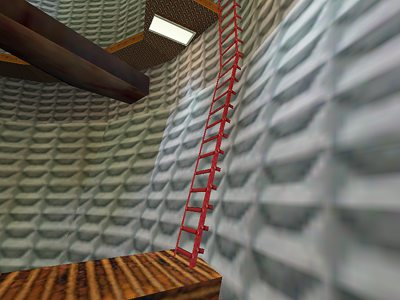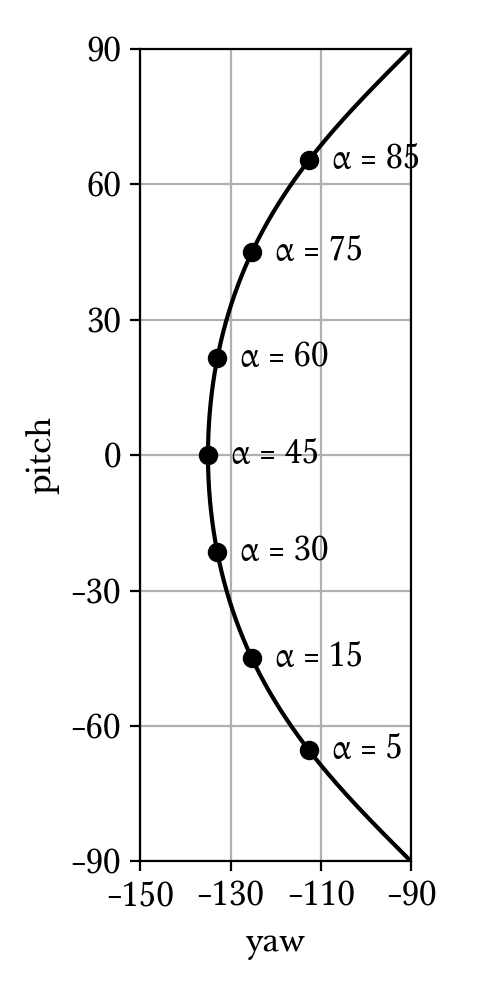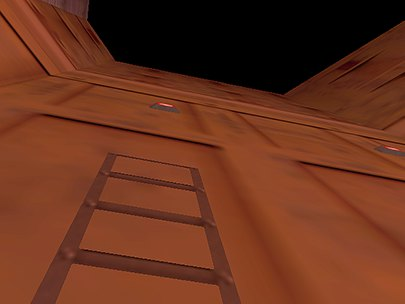Ladders are ubiquitous in Half-Life maps, most of which are perfectly vertical. The climbing speed along a ladder can be increased beyond what the developers intended, and the method is so straightforward that even a beginner speedrunner could pull it off. A sloped ladder is much rarer, and the viewangles to optimise the climbing speed is less obvious. In this chapter, we derive these viewangles for the general case of a sloped ladder.Fig. 9.1. A sloped ladder section in the Blast Pit map of c1a4e, connected to a perfectly vertical section above. What would the optimal climbing viewangles be for this ladder?

## 9.1. Preliminaries¶

Whether a player is on a ladder or not is checked by the PM_Ladder function near the beginning of PM_PlayerMove. If the player is on a ladder, the PM_LadderMove function will be called soon after, which handles the physics of moving on a ladder and set the movetype to MOVETYPE_FLY. This means that the gravity, friction, and other player movement physics described in Player movement basics are completely skipped. Nevertheless, the basevelocity and collision (see Collision) still apply and PM_FlyMove is called to update the player position. The fact that no gravity is exerted under MOVETYPE_FLY is significant, as will be explained in Ladder exit.

We first introduce and , which are analogues of and from the standard movement physics. Issuing +forward adds 200 to , and issuing +back subtracts 200 from it. Thus, when both +forward and +back are issued we have . Similarly, executing +moveright adds 200 to and +moveleft subtracts 200 from it. Note that the value of 200 cannot be modified without recompilation. For ladder physics, it does not matter what and are. If the duckstate is 2, then and in newer Half-Life versions. This is not true for earlier versions such as the one bundled in NGHL. Regardless of viewangles, jumping off ladder always sets

where is the unit normal vector of the ladder’s climbable plane.

If and then

This equation may be called the fundamental ladder equation and forms the basis of any analysis of movement on the ladder.

## 9.2. Optimal angle between u and ^n¶

To optimise the vertical climbing speed, we assume . We further assume that . Now we have

where is the angle between and . is actually rotated by anticlockwise when viewing into the positive direction of -axis. Expanding ,

We conclude that maximises . If , we have .

Knowing the optimal angle is useful for theoretical understanding, but in practice we must be able to calculate the player’s yaw and pitch angles that maximises vertical climbing speed. For ladders that are perfectly vertical the optimal viewangles are trivial to find, but we need explicit formulae for slanted ladders.

## 9.3. Formulae for optimal yaw and pitch¶

Let with the constraint , so that

where

We are concerned with the vertical velocity, . Written in full and simplifying,

(9.1)

where . To maximise this quantity, we compute

Setting them to zero and simplifying, we obtain the following equations respectively

(9.2)
(9.3)

To solve these equations, we begin by assuming and rewriting equation (9.3) as

Eliminating from equation (9.2), we get

Squaring both sides and simplifying gives

(9.4)

Immediately we observe that is required for this equation to have real solutions. We will deal with this in a later section. At this point we are required to take square roots. This is a critical step and we must carefully choose the signs for the numerator and the denominator, as they will determine the quadrant in which resides.

We define three free variables:

• The sign of . Positive if rightward and negative if leftward.

• The sign of . Positive if forward and negative if backward.

• The sign of . Positive if upward and negative if downward.

The motivation is that we want to be able to automatically determine the correct signs for the numerator and the denominator given our choices of the signs of the free variables. This is useful in practice because we often make conscious decisions regarding the directions in which we want to strafe when climbing ladders. For example, we may choose to invoke +forward and +moveleft, or +back and +moveright. In both cases the resulting velocity is identically optimal, and yet the viewangles are different. By declaring the signs of and as free variables, we can choose the strafing directions mathematically by simply setting the correct signs.

Optimal ladder climbing can go in two possible directions, that is upward or downward. Again, the maximum climbing speed does not depend on the direction, though the viewangles do. Hence we declare the sign of as a free variable.

We will now attempt to formulate the final viewangles in terms of these free variables. To begin, we examine Equation (9.1) more closely. We make three observations:

1. We have when and when .

2. We have .

3. We have for .

We start by considering the sign of . Obviously, the right hand side of Equation (9.1) must have the same sign as the . But observe that there are two terms in the right hand side. Therefore, both terms should also be as large as possible in the direction indicated by the sign of . For example, if we choose , then the terms on the right hand side should be as negative as possible, and vice versa.

We will deal with the angle first, which appears only in the second term, so we will assume that the first term has been dealt with (that is, conforming to the sign of while being as large as possible in magnitude). Now, we want

By one of the observations we made, we have and . Also, is always positive. Hence, equivalently we need

And further,

And thus,

Observe that the required signs of and depends on the chosen signs of and respectively, in addition to the sign of . If we look at Equation (9.4) again, notice that the signs of and determine the signs of the numerator and denominator respectively after removing the squares, because for all .

Deriving from Equation (9.4), the formula for the optimal yaw is thus, in all its glory,

(9.5)

We can adopt the same line of attack for the final formula for . Combining Equation (9.3) and Equation (9.4) gives

Note that the positive square root is taken for the cotangent term because we want . This is followed by a simple rewrite:

Here, we only need to determine the sign of the right hand side as a whole, rather than considering the numerator and the denominator separately. The sign of will indicate whether the player should look upward or downward when climbing. Going back to Equation (9.1) again, we assume the second term has been dealt with, in the same way we assumed the first term to have been dealt with when deducing the signs for the optimal yaw. Now we must have

Since the sign of is completely determined by the sign of , the relation is simplified to

And equivalently,

Notice that the sign of plays a role here. In practice, however, is less efficient to compute. Using one of the observations, we see that . So we are done and we can write out the complete formula for the optimal pitch as follows:

(9.6)

The equations (9.5) and (9.6) can be trivially implemented in code to compute the best ladder climbing viewangles. Note that, since the ladder normal is a unit vector, the that appears in both of these equations can alternatively be written as .Fig. 9.2. Plot of the relationship between yaw and pitch by varying ladder slope angle such that . The free variables have values , , and .

## 9.4. Optimal yaw and pitch when nz<0¶

When , the derivatives will never be zero. However, we can observe that increases when decreases. We also note we constrain the range of to while the value of is unrestricted. Hence we can substitute the maximum value into and solve for . It is found to be

We need to determine what the sign of means. Substituting and into the original vertical velocity equation gives

Note that when . Now we can use the similar technique to deduce the required signs of and , which results in

Again, we wrote these formulae so that they give the correct angles given the freely chosen signs of , and .

## 9.5. Optimal yaw and pitch when nz=1¶

Up to this point we have been assuming the normal vector not being vertical. If , then the second term in the bracket vanishes (since VectorNormalize in pm_shared/pm_math.c returns a zero vector if the input, which is , is also a zero vector) instead of being indeterminate, leaving only

thus

which is maximised when . This can be achieved by setting . If then the yaw should be 45 or 135 degrees away from the intended direction, depending on the signs.

We call “exiting a ladder” to mean moving out of a ladder so that the player is no longer on the ladder (as determined by PM_Ladder). This is different from ladder jumping, where the player jumps off a ladder, which has been described in Preliminaries. In some speedrunning context, ladder exit may be referred to as ladder jumping, though for the purpose of this documentation we do not adopt this meaning.Fig. 9.3. A common and old trick in the c1a0e test chamber map, where the player jumps onto the lamp above by exiting the ladder at full speed at a lower frame rate.

Fig. 9.3. illustrates a common use of ladder exit strategy in speedrunning. In the test chamber map, it is desirable to avoid getting teleported to Xen, and one way to avoid this is to jump onto the lamp above to avoid a big trigger_transition below. Interestingly, the lamp is unreachable at higher frame rates, but easily accessible at lower frame rates. This runs counter to the intuition of jumping in Half-Life where the normal jumping height is frame rate independent as explained in Gravity.

To understand this trick, first recall that the movetype is assigned to be MOVETYPE_FLY while on the ladder, which prevents the gravity to act on the player. Suppose in a frame, the player starts off on the ladder with vertical position and is moving away to exit the ladder at some vertical climbing speed . The player position will be updated as per usual by PM_FlyMove.

Suppose the new position is no longer on the ladder and is in the air. Despite this, the new vertical velocity is the same as before and no gravity will be applied until the next frame!

Now consider the next frame. Since the movetype is no longer MOVETYPE_FLY, gravity will act on the player like normal. The game thus computes

And at frame , it can be shown that

Or writing in terms of time ,

Observe that at any time , the vertical velocity is always higher than expected by normal jumping physics by a constant . In addition, the vertical position higher than expected by . This is why the ladder exit strategy in the test chamber works. By lowering the frame rate, the jump height can be increased. For example, at 1000 fps and , the extra height is only . At 20 fps, however, the extra height is . The extra 20 units can make a noticeable difference.

In general, if it is desired to attain as much height as possible by exiting a ladder, possibly with damage boosting immediately afterwards, it is always more optimal to exit the ladder at a lower frame rate.

## 9.7. Non-normal n¶

It may be possible for the ladder normal to not be normalised. This can happen if the player manages to “enter” the ladder entity. This may be achieved by ducking and unducking underneath the ladder entity.